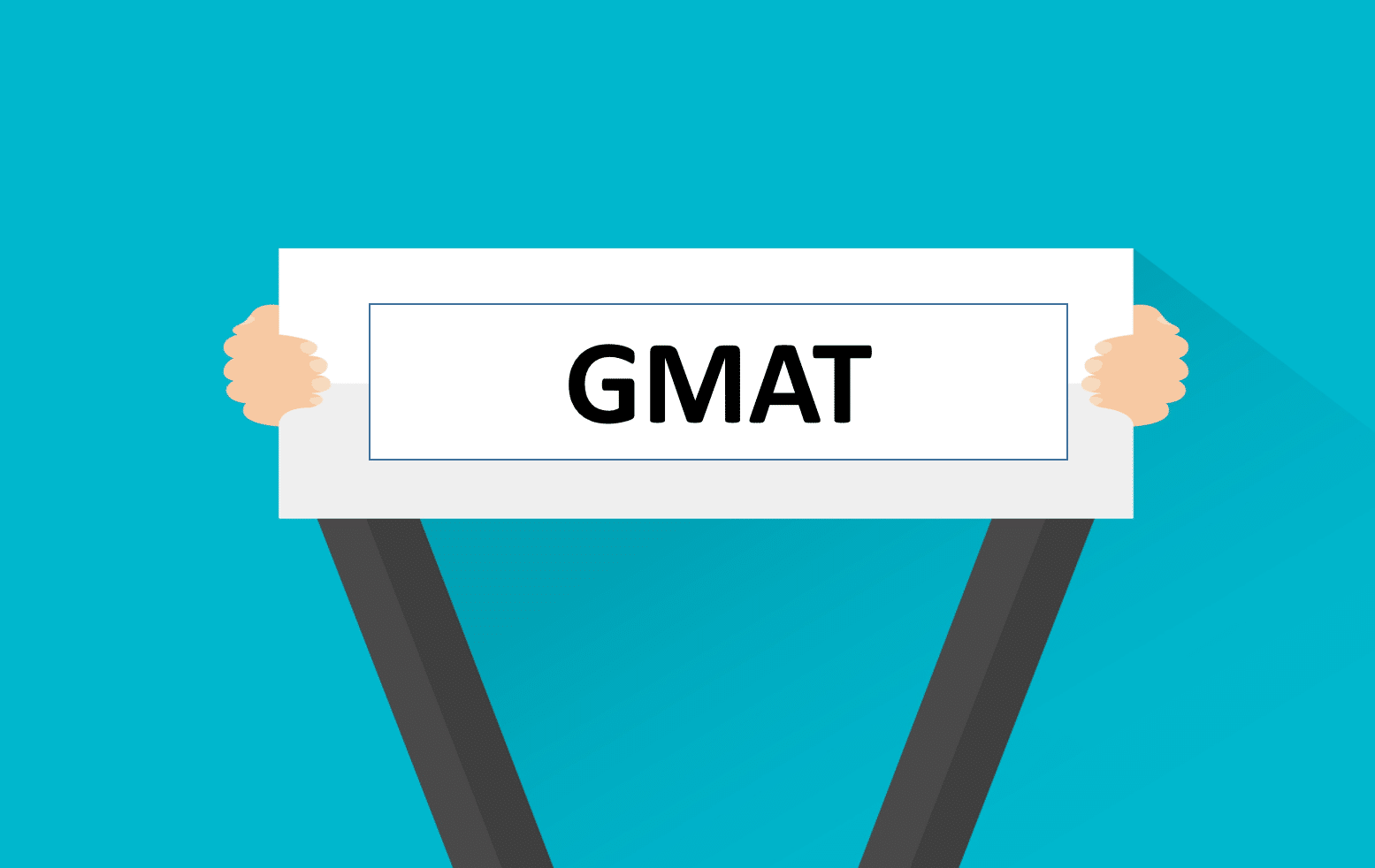## GMAT Quantitative: Tackling Advanced Math ProblemsThe Graduate Management Admission Test (GMAT) is a standardized examination used by business schools worldwide to assess the readiness of candidates for graduate-level management programs. The GMAT Quantitative section evaluates your mathematical skills and problem-solving abilities. While the questions on this section are designed to be challenging, with effective strategies and focused preparation, you can excel in the GMAT Quantitative section. In this guide, we'll explore how to tackle advanced math problems on the GMAT Quantitative section.

## Understanding the GMAT Quantitative Section

Before diving into advanced problem-solving strategies, it's essential to understand the GMAT Quantitative section's format:

### - Question Types:

The GMAT Quantitative section includes two types of questions: Problem Solving (PS) and Data Sufficiency (DS).

### - Problem Solving (PS):

PS questions are conventional multiple-choice math problems that require you to solve for a single correct answer.

### - Data Sufficiency (DS):

DS questions assess your ability to determine whether given data is sufficient to answer a specific question. You won't need to calculate an actual answer; instead, you evaluate the sufficiency of the provided information.

### - Content Areas:

The GMAT Quantitative section covers various math topics, including arithmetic, algebra, geometry, and statistics.

## Strategies for Tackling Advanced Math Problems

### 1. Master Fundamentals First:

- Before attempting advanced problems, ensure you have a solid understanding of fundamental math concepts. Review basic arithmetic, algebra, and geometry principles.

### 2. Learn Problem-Solving Techniques:

- Familiarize yourself with problem-solving techniques commonly tested on the GMAT, such as algebraic manipulation, geometry visualization, and number properties.

### 3. Practice Regularly:

- Consistent practice is key to success. Work through a variety of math problems, including official GMAT practice questions, textbooks, and online resources.

### 4. Time Management:

- Allocate a specific amount of time to each question during practice. This helps you manage time effectively during the actual GMAT.

### 5. Identify Patterns:

- GMAT questions often follow specific patterns or templates. Recognizing these patterns can simplify problem-solving. Practice identifying common question structures.

### 6. Use Alternative Approaches:

- Don't rely on a single approach to solving problems. Develop multiple problem-solving techniques to tackle different question types.

### 7. Solve Real GMAT Questions:

- Official GMAT questions are the best representation of what you'll encounter on the exam. Use official GMAT prep materials to get a feel for the actual test.

### 8. Review Mistakes Thoroughly:

- After completing practice problems, review your mistakes. Understand why you got certain questions wrong and learn from your errors.

### 9. Work on Mental Math:

- Developing strong mental math skills can save time on calculations. Practice mental calculations for addition, subtraction, multiplication, and division.

### 10. Optimize Data Sufficiency Skills:

- Data Sufficiency questions require a unique approach. Learn to analyze information efficiently and make decisions based on the data provided.

### 11. Stay Calm and Move On:

- If you encounter a particularly challenging problem, don't spend too much time on it initially. Mark it for review and move on to easier questions. Return to challenging questions later if time allows.

### 12. Use Scratch Paper Effectively:

- Utilize the provided scratch paper to write down calculations, sketch diagrams, and organize your thoughts. It can help you stay organized and avoid errors.

### 13. Take Practice Tests:

- Periodically take full-length GMAT practice tests to simulate test conditions and assess your overall progress.

## Conclusion

Tackling advanced math problems on the GMAT Quantitative section requires a combination of strong foundational knowledge and effective problem-solving strategies. By following these strategies and dedicating yourself to consistent practice and learning, you can confidently navigate the GMAT Quantitative section and achieve your best possible score. Remember that GMAT success is not solely about answering questions correctly but also about managing time efficiently and maintaining a clear, focused mindset during the test